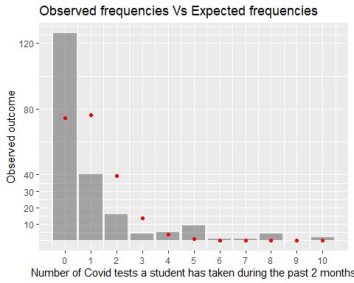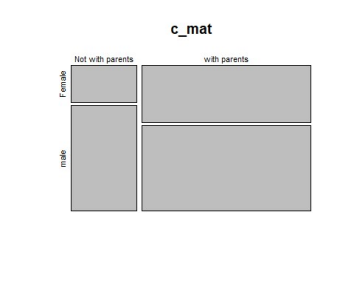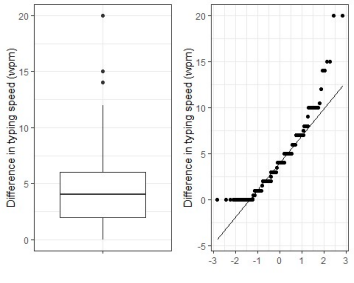# Impact of Covid Crisis On Students

Impact of Covid Crisis On Students This report provides an analysis on the life of students based on the survey data  collected from the cohort of Data2001 and Data2002. With a focus on the impact of  Covid crisis and the life of ordinary student lives.

##### The analysis will be based on 4 aspects:

1. whether the number of COVID tests taken by a student follows a poisson  distribution

2. whether females are more likely to live with their parents than males

3. A test on the population mean of the weekly exercise hours of students

4. Does  the time a student spent on daily exercising agree with result of the 2011-2012 health survey of average daily duration of physical activity collected by the Australian Bureau of Statistics

The class survey consisting of 29 questions gathered 211 responses. This  report mainly focuses on discussing the number of Covid-tests taken by students,  living arrangement and weekly/daily exercise hours of students.

In this report, the missing values that are either defined as NA or empty string were  removed before conducting the relevant tests. The code for cleaning was adapted  from the code of Tarr (2021).

##### Discussion

The survey data was not a random sample of the Data2x02 student. The sample  selection of the survey was not made randomized but voluntary. This has  introduced many biases.

1. Undercoverage bias could be potential as there were students neglecting  important Ed announcements. This made them miss out on the survey. The  variable ‘What year of university are you in?’ could be affected as many of the  first-year students are new to the Ed system. The group was hence not well  represented in the survey.

2. Non-response bias took place as some of the students were inactive to be  involved with the survey. They were more willing to concentrate on personal  matters such as studying, working and socializing

3. Question order bias was introduced by “How do you assess your  mathematical ability” and “How do you self assess your R coding ability?”.  Furthermore, the successive order could have the latter one affected. Studies(1) have  proven people tend to relate a specific question with a general question. In addition, the mathematical ability and R coding ability both assess the learning outcome of  a student. Students who had a weaker background in Math tended to also say  they were weak in R coding to make their answers internally consistent.

The numeric response questions include ‘How tall are you?’ and ‘What do you  believe is the average entry salary in Australian Dollars of a data scientist who has  just completed their undergraduate degree in data science?’ should specify a unit of  measurement to make the responses consistent to improve. Furthermore, the  question ‘How are you finding DATA2002 so far?’ should be answered in numbers  instead of using difficulty level. Different people have different understandings of  the standard of ‘easy’ or ‘difficult’. Answering the question on a scale of 1-10 would  be more appropriate. This allows the survey to collect more expandable responses.

Analysis

Poisson Distribution

In the first section, we look at how has affected the lives of the students with the  data of Covid tests

#### Furthermore, data shows there are 126 students who have taken 0 Covid test during the last 2 months and the detailed table shows below.

Furthermore 0 1 2 3 4 5 6 7 8 10

In addition, 126 40 16 4 5 9 1 1 4 2

Because none of the responses stated that they have taken 9 covid tests we need to  manually add a 0 between 8 and 10 to make the vector length consistent with our expected outcome in the later goodness of fit test.

a = vector(mode = “numeric”,length = 11)

a = as.vector(display)

Furthermore  126 40 16 4 5 9 1 1 4 0 2

In the initial phase of analyzing the number of Covid tests, the missing values from  the variable are removed. We are able to calculate the mean parameter �� which is  1.02 and the expected cell counts as follows.

##### By summing up the occurrences of the numbers from 0-10 in the data, we are able  to visualize the distribution. The observed counts are shown by the bars. While the red dots represent the expected cell counts under the null hypothesis of a Poison  distribution. The number of COVID tests does not follow a Poisson distribution  according to the graph. This is because the expected frequencies and observed  frequencies are less consistent.Test of goodness of fit for a poisson distribution

Hypothesis: The number of COVID tests a student has taken in the past two  months follow a Poisson distribution vs : The number of COVID tests a student  has taken in the past two months does not follow a Poisson distribution

Assumptions: independent observations

The cells where the expected number of counts is < 5 violates an assumption that �� = ����≥ 5 which are 3,6,7,8,10 and need to be combined so �� ≥ 3 = 4 + 5 + 9 + 1 + 1  + 4 + 2 = 26, �� ≥ 3 = 17.8

After combining the columns with the expected number of cell counts < 5, we’re left  with 4 goal outcomes (0, 1, 2, 3 and 3+), the test statistic will follow a chi-squared  distribution with 4−1−1=2 degrees of freedom as we have estimated the mean  parameter ��

Test statistic: �� = ∑(௒ି௘)

௜ୀଵ . Under ��, �� ∼ ��௞ିଵ

Observed test statistic: ��= 70.90525  p-value: ��(��≥ ��) = 0

approximately.

Decision: Since p-value is less than 0.05, we reject the null hypothesis. We conclude  that the data is not consistent with the null hypothesis that the number of COVID  tests a student has taken in the past two months follow a Poisson distribution.

##### Whether females are more likely to live with their parents than males

Males are traditionally considered more independent than females. Moreover, an exploration of whether females are more likely to live with their parents will provide an insight  into the topic. In the meantime, the living environment is an essential topic in  students’ life. Accompanying family members become rather difficult when they are  not living together. A investigation into the topic will give us a deeper  understanding of how students’ lives differ depending on their gender during the  Covid crisis.

During the data cleaning of the gender variable, Non-binary gender is removed as  this analysis is focusing on the differences between males and females.

The responses under living_arrangement(“What are your current living  arrangements?”) are categorized into two types: 1. Living with their parents 2. Not  living with their parents

Let ������ be the probability of an observation falling in the (i,j)th category.  ����. = ∑ ��

௝ୀଵ ���� and ��.�� = ∑ ��

௜ୀଵ ����

Such that ��11 = ��(�� = ������������ℎ��������������, �� = ������������) = ��(�� = ������������ℎ��������������)��(�� = ������������) = (��. 1��1. )

After cleaning we end up with the following table:

Not with parents With parents

Female 15 59

Male 42 87

##### From the mosaic plot, we have an overview of the data. Independence is shown  when the boxes across categories all have the same areas, however they do not have  the same areas in this case as shown from the graph.Test of independence

##### Hypothesis: ��଴: The distribution of whether or not living with their parents is the  same for both gender vs ��ଵ: The distribution of whether or not living with their  parents is not the same for both gender

Assumptions: independent observations and �� = ����≥ 5 The expected cell count  is > 5 which fulfills the assumption where �� = ����≥ 5

Female male

Not with parents 20.8 36.2

with parents 53.2 92.8

Test statistic: �� = ∑ ∑ (௒೔ೕି௘೔ೕ)

௝ୀଵ

approximately.

௜ୀଵ . Under ��, �� ∼ ��(௥ିଵ)(௖ିଵ)

೔ೕ

Observed test statistic: �� =\$2.9338

p-value: ��(��≥ ��) = 0.08674

Decision: Since the p-value = 0.08674 > 0.05, we do not reject H0. There is  insufficient evidence to conclude that the distribution of whether or not living with  their parents is not the same for both gender i.e. The chance of living with parents  do not depend on one’s gender.

Population mean of the weekly exercise hours of students

Apart from the focus on the students’ living environment under Covid, physical  health should also be a center of focus. This is due to the fact that Covid has made  out-door activity less frequent. Studying the time students spend on exercise with  various activity restrictions will provide us with a wider view about the impact of  Covid.

The question “How many hours each week do you spend exercising?” are consisting  of 203 responses after NA values have been removed as we are analyzing based on  existing values.

##### Since exercising 80 hours a week is physically questionable and we are analysing based on the normal population. it is reasonable to remove the outlier.

We can also calculate the mean value and standard deviation of the data that we use for our testing. Mean = 4.38 SD = 3.55

Before we conduct our one sample t test, we shall generate a Q-Qplot and boxplot to  check if the data fulfills the assumption of normal distribution. With its five number  summary (“minimum”, first quartile (Q1), median, third quartile (Q3), and  “maximum”) of the boxplot, we see that the Maximum hours are 20 and its Q1 and  Q3 are sitting in 0-10 we can also interpret this as the majority of the students  taking the survey are exercising 0-10 hours a week. Meanwhile, we see that the  median is roughly in the middle of the box, suggesting the data follows a normal  distribution. The QQ plot on the right also indicates a normal distributionOne sample t test

The Australian government has recommended 2.5 to 5 hours of moderate intensity  physical activity for adults, we can take the mean of 2.5 and 5 to become our  hypothesized value for the one-sample t test. So hypothesized value = (2.5 + 5)/2 = 3.75

##### We want to know whether the mean is statistically different from the value.  Hypothesis: H଴: �� = 3.75 vs Hଵ: �� ≠ 3.75

Assumptions:

��௜௜ௗ ��(��, ��

As our sample is large enough we can safely say that the normality assumption is  satisfied and the graph also indicates a clear normal distribution.

Test statistic:

�� =��− �� ��

√��

∼ ��௡ିଵ ���������� ��

And the degree of freedom are 202-1 = 201  Observed test statistics:

��=��‾ − ��

��

√��

p-value: ��(��ଶ଴ଵ ≤ 2.51) = 0.13  Decision:

qt(c(0.9,0.95,0.975),202)

=4.38 − 3.75 3.55

√202

= 2.51

##  1.285757 1.652432 1.971777

##### At the level of significance a = 0.05, We reject H଴ because test statistic ��0 = 2.51is  larger than the critical value 1.97. We conclude that �� ≠ 3.75

t.test(x\$Stab,mu = 3.75, alternative = “two.sided”)

##

Furthermore ## One Sample t-test

data: x\$Stab

t = 2.5072, df = 201, p-value = 0.01296

Furthermore alternative hypothesis: true mean is not equal to 3.75 ## 95 percent confidence interval:

3.883830 4.869635

Furthermore ## sample estimates:

mean of x  = 4.376733

How many hours a day do students spend doing exercise?

Concluding with the test of the population mean, studying how one’s exercise cycle  has changed will add in the depth of our analysis. Lastly, we can do this by comparing the  survey data with the health survey data in the past year.

##### In order to compare the data from the survey to the result of the 2011-2012 health survey conducted by ABS. We convert the weekly-exercise time to daily-exercise time. Moreover, this is done by dividing the responses under:

‘How many hours each week do you  spend exercising?’ by 7. Furthermore, the data are further categorized into a rough time range. Where category 0-0.5 includes 0 minute to 29 minutes, 0.5-1 includes 30 minutes to  59 minutes, etc. In addition, the average_daily_exercise looks like this after the cleaning steps  are performed.

0 0-0.5 0.5-1 1-1.5 1.5-2 2-2.5 2.5-3 3+

22 67 65 41 1 4 2 1

##### Moreover, by examining the statistics obtained from the health survey, we obtain the following  proportions of daily exercise time for the population aged above 18 and we can see  that most of the population spent about 0-0.5 hours exercising per day in 2011-1012.

Physical activity(%)

0 20.3

0-0.5 39.2

0.5-1 21.4

1-1.5 10.0

1.5-2 3.7

2-2.5 2.0

2.5-3 0.9

3-3.5 0.6

3.5-4 0.2

4-4.5 0.3

4.5-5 0.1

5+ 1.3

##### In the class survey data we do not have any response spending 3-5 hours doing  exercise per day, we therefore combine the proportions of ‘3-3.5’ ‘3.5-4’ ‘4-4.5’ ‘4.5- 5’ ‘5+’ into ‘3+’, so the health survey data will be:

Physical activity(%)

0 20.3

0-0.5 39.2

0.5-1 21.4

1-1.5 10.0

1.5-2 3.7

2-2.5 2.0

2.5-3 0.9

3+ 2.5

Under the circumstance, a chi-square-test will be appropriate, let ���� be the  probability in the �� hours such that �� = 0, 0-0.5,0.5-1,1-1.5,1.5-2,2-2.2.5,2.5-3,3+ We  have two hypothesis:

Null hypothesis: ��= 20.3% ��(଴ି଴.ହ) = 39.2% ��(଴.ହିଵ) = 21.4% ��(ଵିଵ.ହ) = 10.0% ��(ଵ.ହିଶ) = 3.7% ��(ଶିଶ.ହ) = 2.0% ��(ଶ.ହିଷ) = 0.9% ��ଷା = 2.5%

Alternative hypothesis”: The proportions of exercise hours in the class survey do not  follow the model. i.e. at least one equality does not hold

To analyse the hypothesis, we firstly draw a a visualization of the observed outcome  of daily-exercise hour vs the expected outcome of daily-exercise hour from the  health surveys:We see that  in the observed outcome, the observations in 0-0.5 hours are of a similar height as  the expected outcome, this agrees with our hypothesis where ��(଴ି଴.ହ) = 39.2%.  However the observations in 0.5-1 and 1-1.5 hours are significantly smaller in the  expected outcome in comparison to the observed outcome.

##### In our assumptions, ��௜ = ����௜ ≥ 5, however the last three cells have their expected  number of counts less than 5 which violates an assumption. We hence need to  combine the last three cells so the combined outcome fulfills the assumption.

We see the observations for 2+ hours are similar in the observed outcome. But again,  two outcomes are different in 1.5-2 hoursWith the  chisq test, X-squared = 49.824, df = 5, p-value = 1.506e-09 (can be  rounded to 0)

chisq.test(new_y,p= new_dd)

##### Furthermore Chi-squared test for given probabilities

Furthermore X-squared = 49.824, df = 5, p-value = 1.506e-09

Chi-squared goodness of fit test

Hypothesis: ��: ��= 20.3% ��(଴ି଴.ହ) = 39.2% ��(଴.ହିଵ) = 21.4% ��(ଵିଵ.ହ) = 10.0% ��(ଵ.ହିଶ) = 3.7% ��(ଶିଶ.ହ) = 2.0% ��(ଶ.ହିଷ) = 0.9% ��ଷା = 2.5%

��: In addition, the proportions of exercise hour in the class survey do not follow the model.  i.e. at least one equality does not hold

Assumptions: independent observations and �� = ����≥ 5

Test statistic: �� = ∑(௒ି௘)

௜ୀଵ . Under ��, �� ∼ ��௞ିଵ

Observed test statistic: �� = 49.824  p-value: ��(��≥ ��) = 0

approximately.

##### Decision: Since the p-value = 0 < 0.05, we reject H0. There is strong evidence  against in the data against ��଴, the class survey data does not agree with the  proportions introduced by the health survey

From the chi-square test, we perceive that the daily exercise hour of students is no longer consistent with the data obtained from the 2011-2012 Health survey.  Particularly, there are more people exercising more than 0.5 hours a day.

#### Limitations and Conclusion

This report concludes with the findings that 1. The Covid-tests an individual has  taken during the past 2 months do not follow a poisson distribution 2. Gender does  not affect the chance that a student is living with their parents 3. The daily exercise  cycle of students have changed since 2011-2012

Apart from the biases discussed in the introduction, the main limitation comes from  the question ‘How many hours each week do you spend exercising’, the result after  converting the numeric into a specific range (e.g. 1.2 hours to 1-1.5 hours range)  might be inaccurate as requiring a numeric response can be too strict for this type of  question. Instead, range can reduce potential inaccuracies caused by strict standards.

Appendix

abstract/55/1/3/1819909?redirectedFrom=fulltext NORBERT SCHWARZ, Furthermore, FRITZ  STRACK, HANS-PETER MAI, ASSIMILATION AND CONTRAST EFFECTS IN PART WHOLE QUESTION SEQUENCES: A CONVERSATIONAL LOGIC ANALYSIS, Public  Opinion Quarterly, Volume 55, Issue 1, SPRING 1991, Pages 3–23,  https://doi.org/10.1086/269239